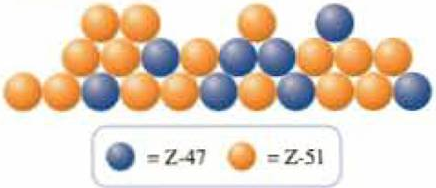Chapter 2, Problem 2.56QPGeneral Chemistry - Standalone boo...

11th Edition
Steven D. Gammon + 7 others
ISBN: 9781305580343

Solutions

Chapter
SectionGeneral Chemistry - Standalone boo...

11th Edition
Steven D. Gammon + 7 others
ISBN: 9781305580343
Textbook Problem

While roaming a parallel universe, you discover the hypothetical element “Z.” You obtain a representative sample of the element and discover that it is made up of two isotopes, Z-47 and Z-51. To help your science team calculate the atomic weight of the substance, you send the following drawing of your sample with your report.In the report, you also inform the science team that the blue atoms are Z-47, which have an isotopic mass of 47.621 amu, and the orange atoms are Z-51, which have an isotopic mass of 51.217 amu. What is the atomic weight of element Z?

Interpretation Introduction

Interpretation:

The atomic weight of element “Z” has to be calculated.

Concept Introduction:

The elements that occur may have isotopes.  Hence, the average of the atomic mass for the naturally occurring element is considered and it is expressed in atomic mass units.  Atomic weight is also referred as atomic mass.  Actually atomic weight simply means it is “average atomic mass” only.

Explanation

The isotope Z-47 has isotopic mass of 47.621 amu .

The isotope Z-51 has isotopic mass of 51.217 amu .

In the given figure in the problem statement, there are eight blue spheres and sixteen orange spheres.  Blue spheres represent Z-47 isotope and orange spheres represent Z-51 isotope.

To find the atomic weight of the element, the average of the isotopic mass has to be found out.

Totally twenty four spheres are present in the given figure.

824(47.621 amu) + 1624(51.217amu) = 15

Still sussing out bartleby?

Check out a sample textbook solution.

See a sample solution

The Solution to Your Study Problems

Bartleby provides explanations to thousands of textbook problems written by our experts, many with advanced degrees!

Get Started

Find more solutions based on key concepts